#### How to Create a Bitcoin Mining Calculator in Excel for Profitability

Bitcoin mining can be a profitable venture, but it is important to calculate the profitability of your mining operation before you get started. This can be done by using a Bitcoin mining calculator.

A Bitcoin mining calculator is a tool that can help you estimate the profitability of your mining operation based on a number of factors, including the price of Bitcoin, the cost of electricity, and the hashrate of your mining rig.

To create a Bitcoin mining calculator in Excel, you will need the following:

• The current price of Bitcoin
• The cost of electricity per kilowatt-hour
• The hashrate of your mining rig

Once you have gathered these materials, you can follow these steps to create your Bitcoin mining calculator: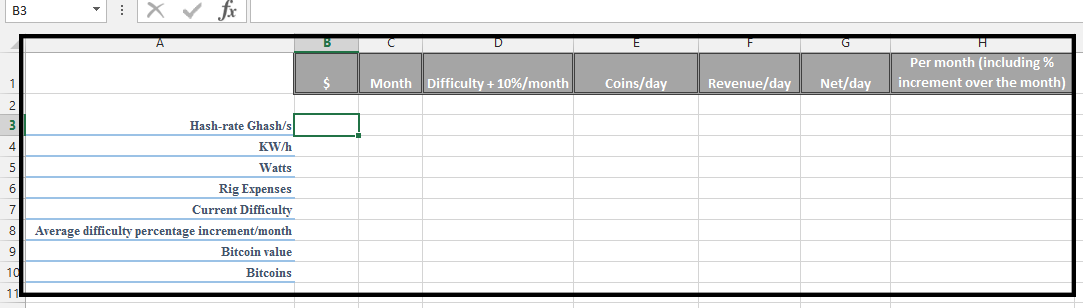Put the price that you know of under the \$ column.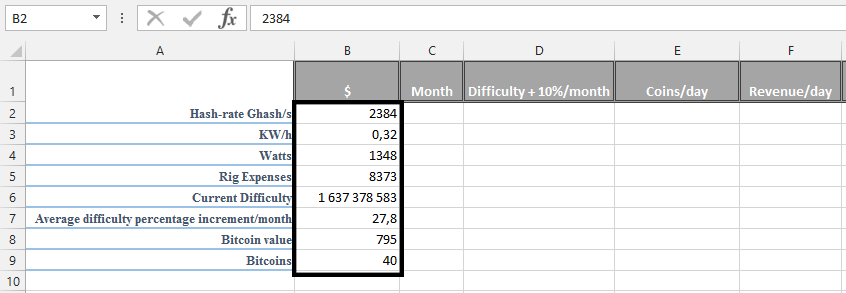Label the months.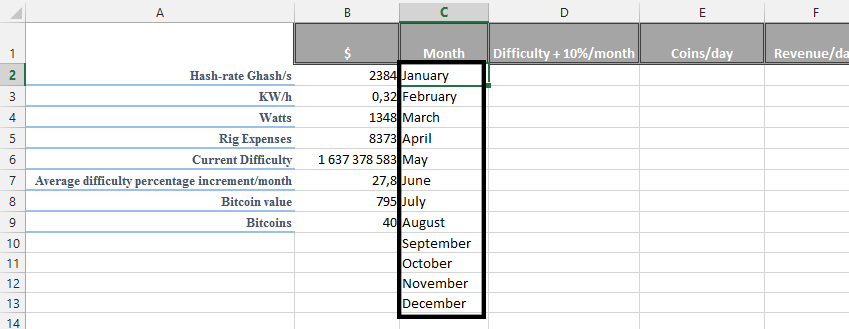In the D column, under Difficulty + 10%, and place the same value you put beside the Current difficulty in the previous step. In this case, it’s (=B6).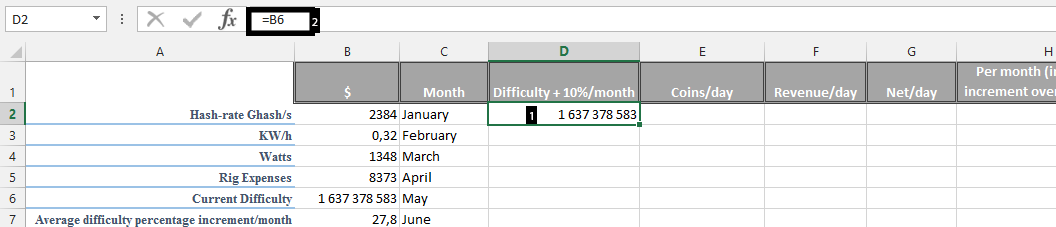## Preparing Bitcoin mining calculator

Click under the result and type =D2+(D2*(\$B\$7/100)). Double click on the small square.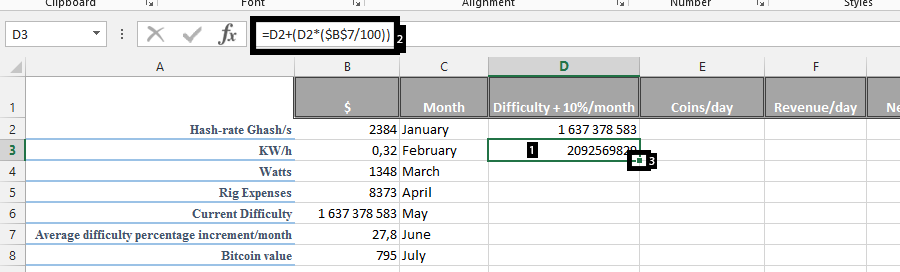Click under Coins/day, and type =\$B\$9*(86400/(D2*((2^32)/(\$B\$2*1000000000)))).

`Note: 86400 is the number of seconds in a day.`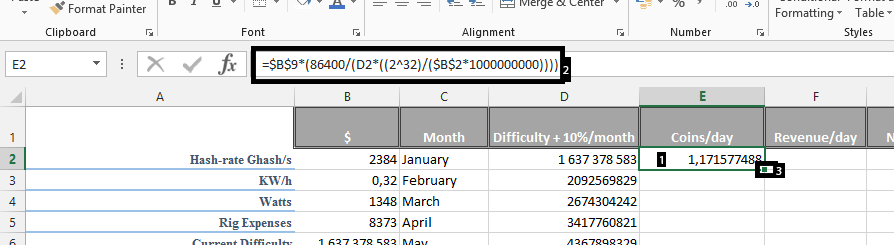Click under revenue/day. Type =E2*\$B\$9.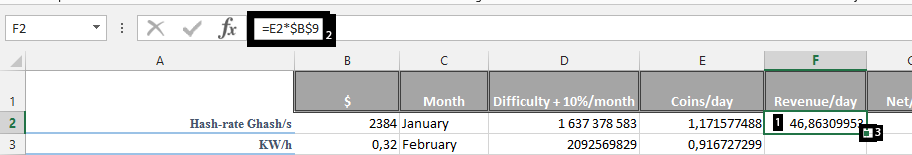Click under net/day (1). Type =F2-(\$B\$3*(\$B\$4/1000)*24).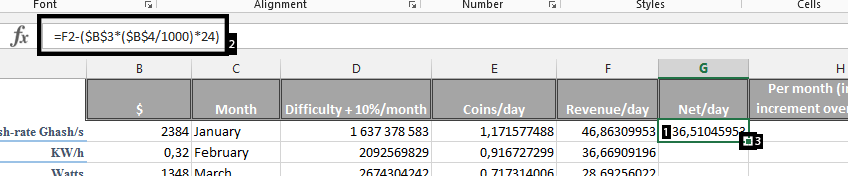Click under Per month (H) column. Type =SUMPRODUCT(–(G2-((G2-G3)/30)*ROW(INDIRECT(1&”:”&30)))).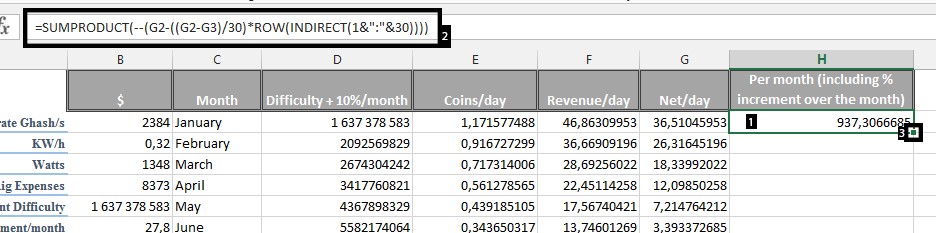Select the empty cell in column G and write Net Annual (1). Then beside it on (H) and type =SUM(H2:H13)-B6.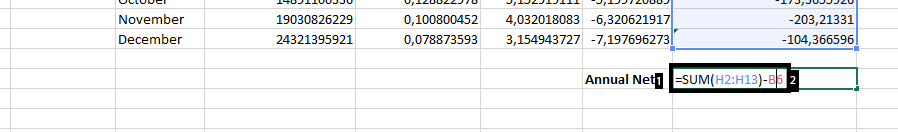You’ve now created a basic Bitcoin mining calculator in Excel. This calculator helps estimate your mining profitability considering factors like hashrate, electricity costs, and Bitcoin’s current price. You can adapt this calculator for other cryptocurrencies like Monero and other AltCoins as well.